Try it now!

USD
10

# The Influence of the Exchange Rate of US Dollars on the Efficiency of Mutual Funds in China - Part 4

1975

## CHAPTER 3 – RESEARCH DESIGN

### 3.1 Introduction

This chapter will present the methodology design employed in this dissertation, for examining the research question. The research design that will be utilized is the t-test, also known as the Student’s t-test. This chapter will explore this research design, providing a theoretical background and the practical application for the actual study.

Have no clue where to find the information for another report that you have to write? Perhaps, it would be better to buy a custom written work at Pro-Papers report writing service for little money and acquire some more spare time?

### 3.2 Theoretical Background on Z-Test

The Z test - test the significance of difference between two values. The Z test can be used to determine whether the value of the correlation coefficient is statistically significant with significance level of 0.05. The Kendall tau-a correlation coefficient:

• Null hypothesisH0: τKen, a = 0 (nocorrelation)
• AlternativehypothesisH1:τKen, a <> 0 (correlation exists)

The formula used to calculate the parameter of the Z test for the correlation coefficient Kendall tau-a is:, where the variation is given by:## 3.3 Theoretical Background on T-Test

When W.S. Gosset developed the t-test he was writing under the pen name “Student”. This is why, this statistical examination remained known as the Student’s t-test (Pagano, 2009, p. 319). The t-test represents a statistical method of examination, focused on analyzing two population means (Fiserburn, 1977, pp.116-126). T-test is utilized in the statistical investigations that analyze two different groups of samples, and the purpose of applying t-test in a statistical investigation is to examine whether the two analyzed groups are different. The t-test is employed in statistical researches when the variances of the normal distributions of the two analyzed groups are unknown and it usually used in comparing small size samples (Investopedia, “T-Test”, n.d.).

Pagano, on the other hand, observes that t-test is utilized for testing the hypothesis that involve single sample experiments (2009, p. 339). T-test evaluates the distribution of a group, based on the data retrieved from another group, wherein the two statistical groups are in close relation to each other.

This test requires a disposal method that consists in comparing the disparate x0value with the average value, corresponding to the other n-1 values ​​in the sequence of results, being applied for a string of values ​​n <100. In this case, the calculation of the average , is done with the formula:It is also necessary to calculate the standard deviation, neglecting the disparate value x0 (for n-1 values). Next, is determined the ratio: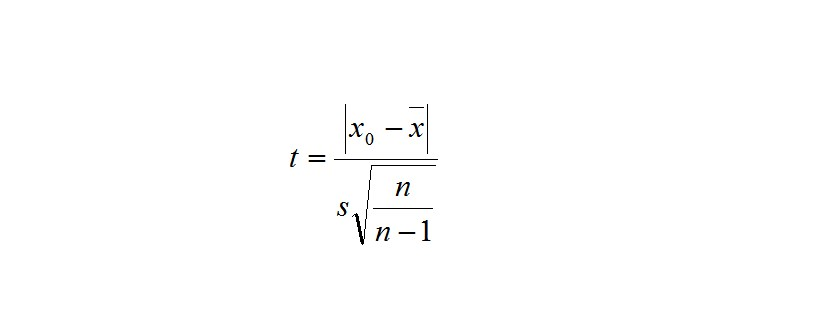(Brennan, 1979, p.32)

The most important aspect is the fact that the size indicators obtained during a research that involves repeated measurements have the same unit as those that were obtained in a cross-type design. For example, in a cross-type design, the emphasis is on highlighting the differences between the two groups versus their joint variability, highlighted by a combined standard deviation. In contrast, in an intra-group design, the emphasis is on highlighting the changes between pre-test and post-test; the standard deviation is also a standard deviation of the changes and not a standard deviation of the recorded raw scores.

## 3.4 Practical Application of T-Test

In the current research, t-test will be applied on two statistical groups: (1) the yuan-dollar exchange rate evolution and (2) the Chinese mutual funds’ performances. In order to analyze whether the yuan – dollar exchange rate has a positive influence upon the efficiency of the mutual funds development in China, there should be initially analyzed the exchange rate. This will be possible by retrieving the annual yuan – dollar exchange rate from the last 14 years. Therefore, the period that will be subjected to analyze starts with 1997, the year when the first mutual fund was developed in China, until this year.

What is contained in the two studied groups, are independent samples, meaning that the samples included in a group are not part of the other group also (Urdan, 2005, p. 89). Therefore, the data contained in the yuan – dollar exchange rate group will not be included in the mutual funds in China group and vice-versa.

In practice, there will be identified the yuan – dollar exchange rate starting with 1997 to 2011, searching the web. The identified data will be inserted in an excel file. Likewise, the values of the mutual funds in China will be also searched for on the web and included in the same table. With these two groups formed, there should be next applied a function that would calculate the probability rate that the exchange rate to influence positively the mutual funds.

The Hypothesis

The working hypothesis for the present study states that the exchange rate evolution has a direct influence over the values of the Chinese mutual funds. In economical terms, this will be translated as: after the opening of the Chinese mutual funds to the international market, the interdependencies and also the associated risks of the mutual funds have become more dependent on the US economical trend. This may be also an expression of the economical “war” that currently is being fought by the two super-powers.

The first set of data that will be used is presented in Table 1 and represents the fluctuation of the exchange rate between the US dollar and the Yuan.

 Year 1997 1998 1999 2000 2001 2002 2003 2004 2005 2006 2007 2008 2009 2010 CNY/USD 8.32 8.3 8.28 8.28 8.28 8.28 8.28 8.28 8.19 7.97 7.61 6.95 6.83 6.77

As already mentioned, the stability of the exchange rate is striking and needs no statistical evidence. However, an initial analysis is needed. The economic growth in China is very much based on exports, the only problem for this country is that its exports are often preferred to those from the EU or Japan for example, mainly due to lower prices and not because innovation or high quality. In part, this is also the results of the actions took by the Chinese authorities to control credit growth, in order to avoid bad loans and the creation of financial bubbles, such as those that affected most developed countries. However, in this way, China has closed the possibility for the Chinese people to invest so that country can have an economy based on investments, and thus competitive. The disadvantage is here that on one hand China’s massive savings power and its economic background allows this country to continue the depreciation of the CNY (Chinese yuan) against the USD dollar) and thus to secure its much needed economic growth.This policy is extremely visible in Table 1, starting with 2004, and having an accentuated movement during the years of the economic crisis (2008, 2009).

The second range of data is presented in Table 2, and represents the global values of the Chinese mutual funds.

 Year 1997 1998 1999 2000 2001 2002 2003 2004 2005 2006 2007 2008 2009 2010 Mutual Funds in RMB 10.8 57.4 84.6 82.1 120.5 256.1 324.6 469.1 856.5 3275 1940 2680 3684

The first thing needed to test is whether the mutual fund’s distribution is normal or not.

The normal distribution is a distribution law of a value around its average. A variable t, follows a normal distribution if the frequency function is represented by the formula:The graphical representation of f(x) is the one presented in Figure 1 and it is called the normal curve or the Gauss bell.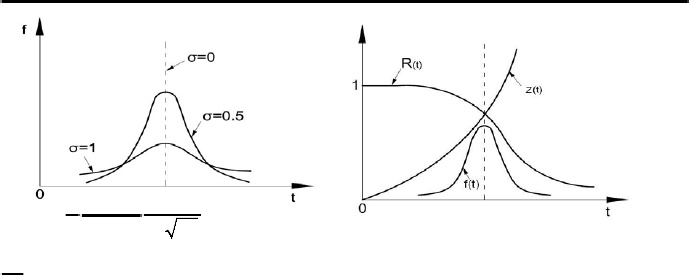Figure 1 – Normal curve (Rubinstein, 1973, p.120)

A normal distribution (Gaussian) of the random error of a variable x is characterized by the fact that the absolute errors, (x- average value of the variable x), of the same module, have the same frequency of occurrence with the plus sign and with the minus sign and as the module of errors is higher they occur more rarely. Such behavior is described by a distribution function that has the form (Gauss):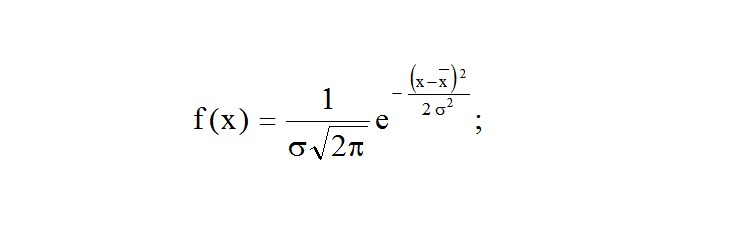called the probability density, the constant s is called the mean square deviation (standard deviation). As Leland states, the significance of the function f (x) is: if ​​a set of N measurements is done (N large enough), then the probability to obtain Ni results between xi and xi+D x is given by: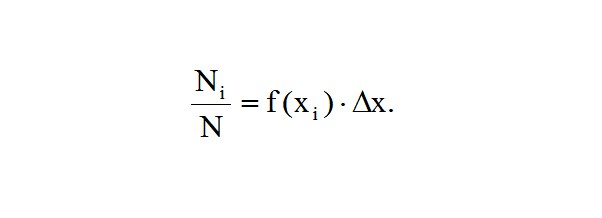(Leland, 1993, p.43)

To the limit the relation for and becomes: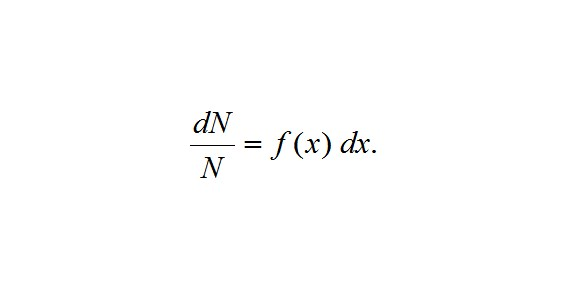The probability of finding ​​x values in the interval [x1, x2] or absolute deviations (errors) in the interval [x1 - x2 -] is given by which corresponds to the shaded area on Figure 2 and the number of deviations from this period is: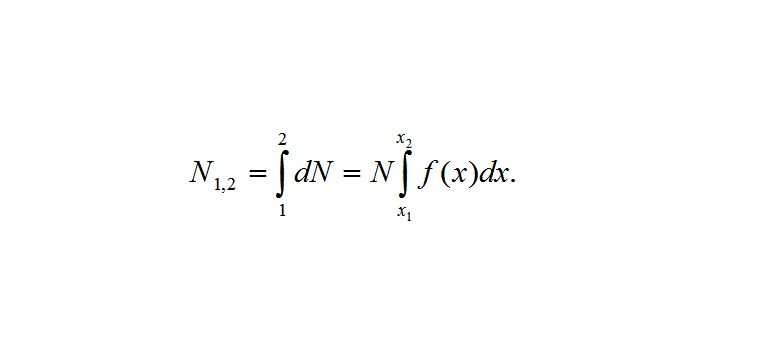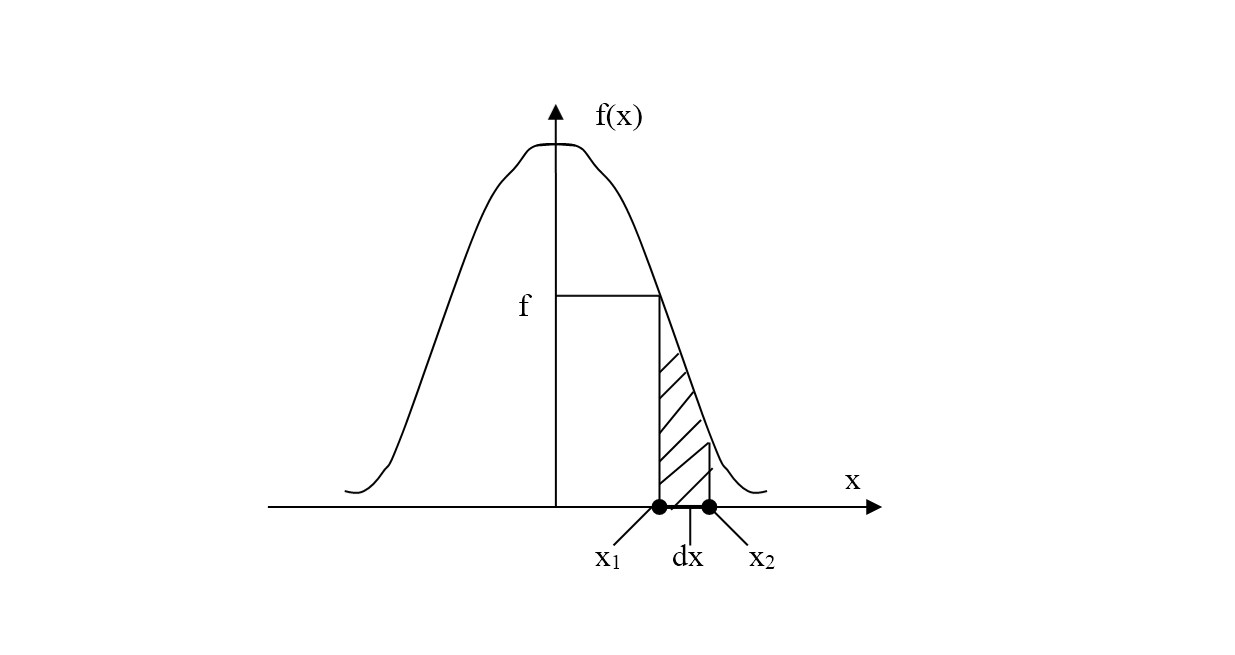Fig. 2

In order to test the normal distribution, the most common method is represented by the calculation of the Skewness and Kurtosis coefficients. The standard deviation and the dispersion are indicators that give a measure of the dispersion of the values ​​in a frequency distribution. In a sense, they provide a measure of the "width" of the distribution (Levitt, 2002, p.11). There are several methods for measuring distortion. The most commonly used is: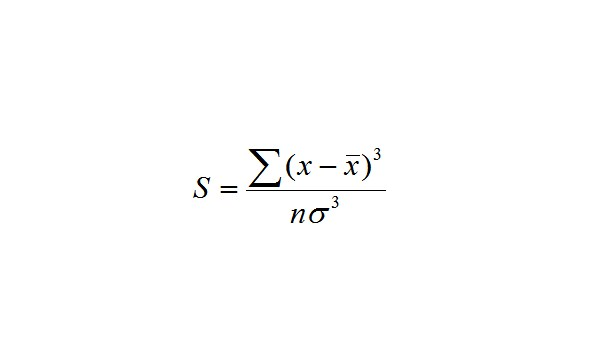, where in the numerator we have cubic deviation from the mean values ​​and s is the standard deviation (Sortino, 2002, p. 31).

We have the following situations:

• ifS< 0than the distortion isnegative;
• ifS > 0 than the distortion is positive.

Kurtosis gives a measure of the expansion toward the values that are concentrated in one part of the frequency distribution. If a class in a frequency distribution contains much of the values from the distribution, than the distribution shows a high degree of kurtosis, and the shape is more pointed. In a distribution with a low degree of kurtosis (plane distribution), each class contains a similar proportion of the values. The formula used for K is;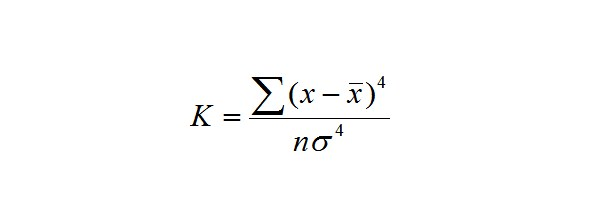(Pedersen & Satchell, 2002, p.23)

Looking for a list of good topics for an argumentative essay that may inspire you for your own brilliant idea? Pro-Papers provides a few lists of argumentative topics for various cases in its blog, so feel free to get acquainted with it.

For normal distribution we have K = 3, for a sharp distribution K> 3, and for a plane distribution K <3.

The null hypothesis for the present study states that there is a direct relation between the exchange rate and the performances of the Chinese mutually funds. The alternative hypothesis, states that there are also other elements that influence the performances of the mutual funds, in a greater amount that the exchange rate between the US dollar and the yuan.Leave a Reply